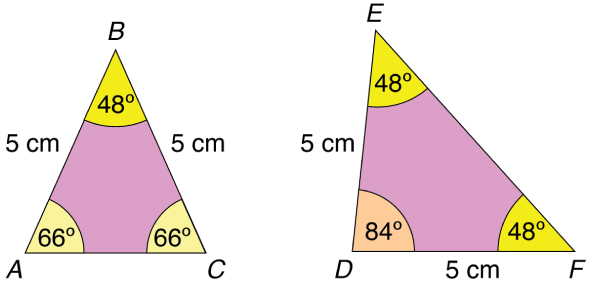# How Much Do You Know About Triangles? Take The Trivia Quiz To Find Out!!

Approved & Edited by ProProfs Editorial Team
At ProProfs Quizzes, our dedicated in-house team of experts takes pride in their work. With a sharp eye for detail, they meticulously review each quiz. This ensures that every quiz, taken by over 100 million users, meets our standards of accuracy, clarity, and engagement.
| Written by Bmahas
B
Bmahas
Community Contributor
Quizzes Created: 1 | Total Attempts: 309
Questions: 10 | Attempts: 309SettingsDid you pay good attention in your Maths class? Do you know everything there is to know about triangles? Can you tell the difference between an acute, obtuse, and right-angled triangle? Take the online quiz to see how much you know and learn more. .

• 1.

### What is the name for a triangle that has all three sides the same length?

• A.

Scalene

• B.

Isosceles

• C.

Equilateral

• D.

Obtuse

C. Equilateral
Explanation
An equilateral triangle is a type of triangle that has all three sides of the same length. In this triangle, all angles are also equal to 60 degrees. This makes it a special type of triangle with symmetrical properties.

Rate this question:

• 2.

### How would you name this triangle by its sides?

• A.

Scalene

• B.

Isosceles

• C.

Equilateral

• D.

Acute

B. Isosceles
Explanation
An isosceles triangle is a triangle that has two sides of equal length. In this case, since the question does not provide any information about the lengths of the sides, we can assume that two of the sides are equal. Therefore, the correct answer is "Isosceles."

Rate this question:

• 3.

### How would you name this triangle by its angles?

• A.

Scalene

• B.

Right

• C.

Obtuse

• D.

Acute

C. Obtuse
Explanation
This triangle would be named "Obtuse" based on its angles. An obtuse triangle is a triangle that has one angle greater than 90 degrees. Since the question does not provide any information about the angles of the triangle, we can assume that it has at least one angle greater than 90 degrees, making it an obtuse triangle.

Rate this question:

• 4.

### How many degrees do the three angles of a triangle add up to?

• A.

90

• B.

180

• C.

200

• D.

360

B. 180
Explanation
The three angles of a triangle always add up to 180 degrees. This is a fundamental property of triangles in Euclidean geometry. It can be proven using the fact that the sum of the angles in any polygon is equal to (n-2) * 180 degrees, where n is the number of sides of the polygon. Since a triangle has three sides, the sum of its angles is (3-2) * 180 = 180 degrees.

Rate this question:

• 5.

### If the three angles of a triangle measure 60, 80, and 40 degrees what type of triangle is it?

• A.

Acute

• B.

Obtuse

• C.

Right

• D.

Straight

A. Acute
Explanation
The three angles of a triangle measure 60, 80, and 40 degrees. In a triangle, the sum of all angles is always 180 degrees. Since the sum of these three angles is less than 180 degrees (60 + 80 + 40 = 180), the triangle is an acute triangle.

Rate this question:

• 6.

### If the sides of a triangle are 8 ft, 4 ft, and 5 ft what type of triangle is it?

• A.

Scalene

• B.

Isosceles

• C.

Equilateral

• D.

A. Scalene
Explanation
A scalene triangle is a triangle in which all three sides have different lengths. In this case, the given triangle has sides measuring 8 ft, 4 ft, and 5 ft, which are all different lengths. Therefore, it can be classified as a scalene triangle.

Rate this question:

• 7.

### If two of the angles in a triangle are 50 degrees and 100 degrees what is the third angle?

• A.

10 degrees

• B.

50 degrees

• C.

90 degrees

• D.

30 degrees

D. 30 degrees
Explanation
The sum of the angles in a triangle is always 180 degrees. If two of the angles are 50 degrees and 100 degrees, the sum of these two angles is 150 degrees. To find the third angle, we subtract the sum of the two known angles from 180 degrees. Therefore, the third angle is 180 - 150 = 30 degrees.

Rate this question:

• 8.

### What type of triangle is shown below?

• A.

Equilateral, Right

• B.

Isosceles, Obtuse

• C.

Isosceles, Right

• D.

Equilateral, Acute

D. Equilateral, Acute
Explanation
The given triangle is an equilateral triangle because all three sides are equal in length. Additionally, the triangle is acute because all three angles are less than 90 degrees.

Rate this question:

• 9.

### What is the name for a triangle that has a 90 degree angle?

• A.

Acute Triangle

• B.

Obtuse Triangle

• C.

Right Triangle

• D.

Isosceles Triangle

C. Right Triangle
Explanation
A triangle that has a 90 degree angle is called a right triangle. In a right triangle, one of the angles is a right angle, which measures 90 degrees. This means that one of the sides of the triangle is perpendicular to the other two sides.

Rate this question:

• 10.

### What makes a triangle Isosceles?

• A.

All three sides are the same length

• B.

Two of the sides are the same length

• C.

None of the sides are the same length

• D.

Four sides are the are the same lengthBack to top